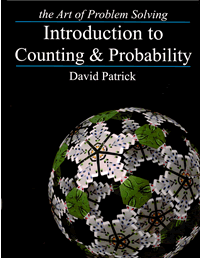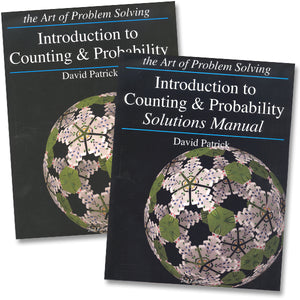# AoPS Introduction to Counting and Probability Text and Solution Set

Vendor
AoPS
Regular price
Sold out
Sale price
\$45.00
Quantity must be 1 or more

Overview

Learn the basics of counting and probability from former USA Mathematical Olympiad winner David Patrick. Topics covered in the book include permutations, combinations, Pascal's Triangle, basic combinatorial identities, expected value, fundamentals of probability, geometric probability, the Binomial Theorem, and much more.

The text is structured to inspire the reader to explore and develop new ideas. Each section starts with problems, so the student has a chance to solve them without help before proceeding. The text then includes solutions to these problems, through which counting and probability techniques are taught. Important facts and powerful problem solving approaches are highlighted throughout the text. In addition to the instructional material, the book contains over 400 problems. The solutions manual contains full solutions to all of the problems, not just answers.

This book is ideal for students who have mastered basic algebra, such as solving linear equations. Middle school students preparing for MATHCOUNTS, high school students preparing for the AMC, and other students seeking to master the fundamentals of counting and probability will find this book an instrumental part of their mathematics libraries.

Diagnostics
Excerpts
Text ISBN: 978-1-934124-10-9
Solutions ISBN: 978-1-934124-11-6